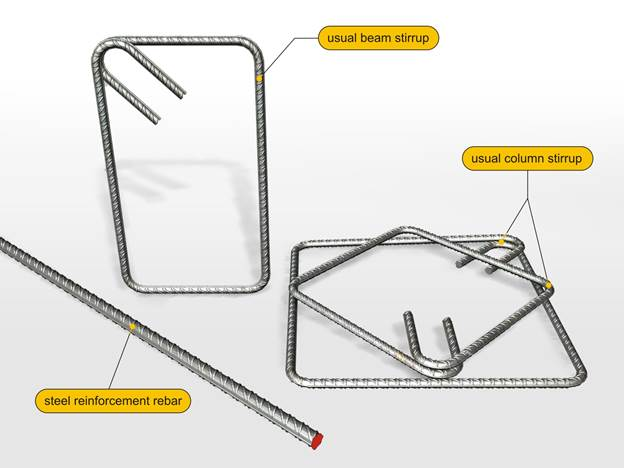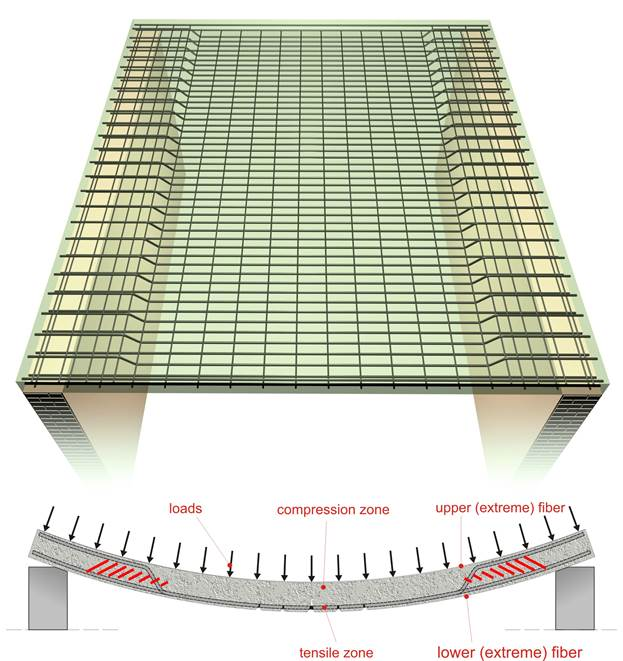# SlabsIntroduction >
The structural frame
The construction
The reinforcement I
The reinforcement II
Quantity/Cost estimation
Detailing drawingsIntroduction >
Wind and Seismic Forces >
Structural model and Analysis
Modelling slabs
Slabs
Seismic behavour of frames
Appendix A
Appendix B
Appendix C
Appendix DIntroduction >
Materials
To be continued >

## Steel reinforcementReinforced concrete is made of two materials, concrete and reinforcement. The reinforcement is usually made out of steel and, rarely, at least for the time being, it is made out of composite materials, composites.
The reinforcement is divided into two basic categories: (a) the longitudinal reinforcement in the form of rebars and (b) the transverse reinforcement mainly in the form of stirrups.

## Behaviour and reinforcement of an one-way slabUnder loading (such as self weight, marble floor coverings, human activities etc) and due to their elastic behaviour, slabs are deformed as shown at the following figure. The real deformation is in the order of millimeters and although it is not visible to the human eye it always has this form. Concrete has a high compressive strength; therefore in the compression zone where there are only compressive stresses, no longitudinal reinforcement is placed. On the contrary, concrete has a very low tensile strength therefore in the slab’s tensile zone where there are only tensile stresses, longitudinal reinforcement is placed.